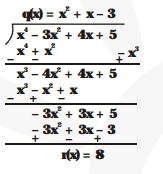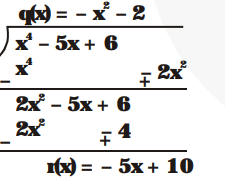# Divide the polynomial p(x) by the polynomialQuestion.

Divide the polynomial p(x) by the polynomial g(x) and find the quotient and remainder in each of the following :

(i) $\mathrm{p}(\mathrm{x})=\mathrm{x}^{3}-3 \mathrm{x}^{2}+5 \mathrm{x}-3, \mathrm{~g}(\mathrm{x})=\mathrm{x}^{2}-2$

(ii) $p(x)=x^{4}-3 x^{2}+4 x+5, g(x)=x^{2}+1-x$

(iii) $\mathrm{p}(\mathrm{x})=\mathrm{x}^{4}-5 \mathrm{x}+6, \mathrm{~g}(\mathrm{x})=2-\mathrm{x}^{2}$

Solution:Hence, Quotient $q(x)=x-3$ and Remainder $r(x)=7 x-9$

(ii) $x^{2}-x+1$Hence, Quotient, $q(x)=x^{2}+x-3$

and remainder, r(x) = 8

(iii) $-x^{2}+2$Hence, Quotient, $q(x)=-x^{2}-2$

Remainder, $r(x)=-5 x+10$